# pandas Series¶

xlSlim was designed to support pandas Series efficiently while keeping the interaction with Excel as intuitive as possible.

Note

Type hints are essential for xlSlim to recognise pandas Series passed between your functions.

Warning

pandas functionality requires a premium licence. See Licensing

## Creating pandas Series¶

pandas Series can be created in three ways:
• within a Python function
• from an Excel range
• from an Excel range of numbers (optimised)

### pandas Series from a Python function¶

This Python code defines a function create_random_ds() that returns a Series of random numbers between 0 and the number of rows, the index is a series of integers from 0 to the number of rows.

```import pandas as pd
import numpy as np
from typing import List, Optional

def create_random_ds(rows: Optional[int] = 1) -> pd.Series:
"""Create a Series of random integers"""
return pd.Series(np.random.randint(0, rows, size=(rows,)), list(range(rows)))
```

Note

All the Python code and Excel files shown are available from github in the xlslim-code-samples repo. I highly recommend downloading the samples from github. The Excel workbooks contain many tips and tricks.

Save the Python code as a new file on your PC. I saved the file in my Documents folder.

Open Excel and enter this `RegisterPyModule()` formula (amending the location to match where you saved the file):

```=RegisterPyModule("c:\users\russe\documents\Series.py","C:\Users\russe\anaconda3\envs\py37")
```

Note

pandas and numpy are not available in the Python installation bundled with xlSlim. The Python module must be registered with an existing Python installation that has numpy and pandas installed.

If we call the create_random_ds() function we see that the result is automatically cached and a cache handle is returned to Excel.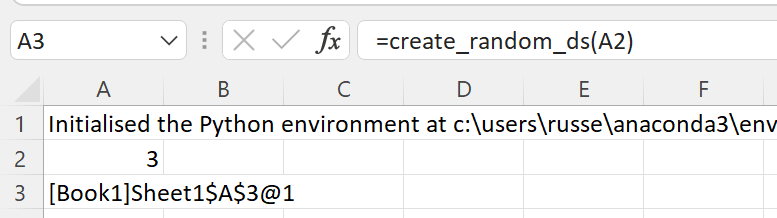The xlSlim function `ViewPyObject()` can be used to view any Python object stored in the memory cache.

```=ViewPyObject(A3)
```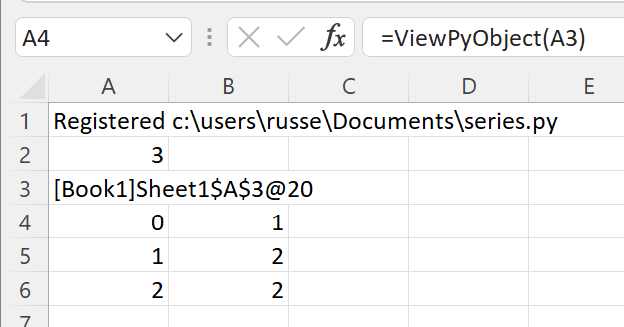### pandas Series from an Excel Range¶

Any two column Excel range can be used to create a pandas Series. The first column is assumed to be the index and the second column is assumed to be the data. This does prevent the use of advanced indexing, Series with advanced indexing should be created within Python functions as shown above.

Add this describe_ds() function to the Python code and re-register the module with `RegisterPyModule()`

```def describe_ds(x: pd.Series) -> pd.Series:
"""Describe this Series."""
return x.describe()
```

This function describes the pandas Series passed in, we’ll use it to show how xlSlim creates pandas Series directly from Excel if the Python function has a type hint for pd.Series.

We create a table of strings and numbers in Excel and pass this to the describe_ds() function.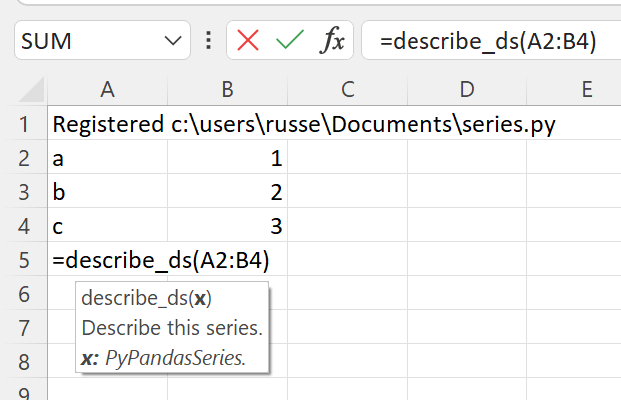The function returns a cached object handle to Excel.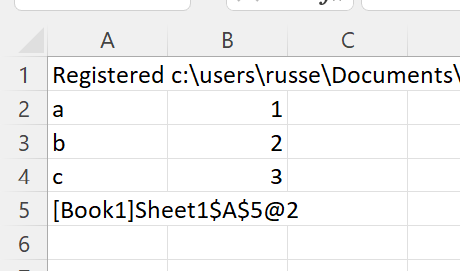A few things have happened here. xlSlim created a pandas Series from the range A2:B4 before passing the data to the Python code. The Python code received a regular pandas Series as expected. The Python function describe_ds() then returned a new pandas Series. xlSlim automatically put this pandas Series into a memory cache and returned the cache handle to Excel.

Using `ViewPyObject()` we can see the Series has been created and passed around as expected.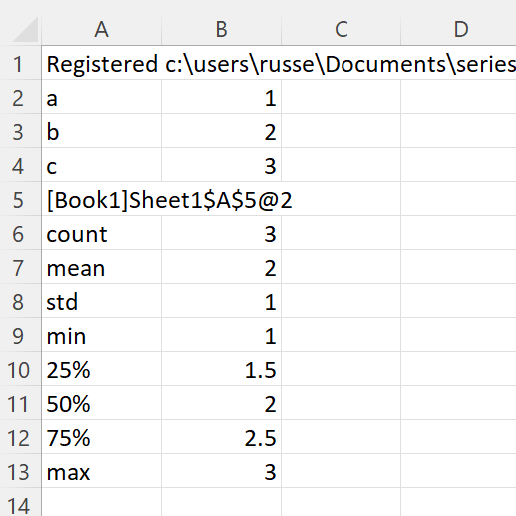### pandas Series from an Excel Range of numbers¶

xlSlim is optimised to create pandas Series from Excel ranges of numbers. Ranges of numbers are converted to pandas Series very efficiently with only direct memory copies being used.

xlSlim cannot determine if the Excel range passed in only contains numbers (Technically it could but this would be very slow). Therefore xlSlim creates a special version of every Python function that expects one or more pandas Series - these special functions have the same names with “_num_only” appended.

Let’s create a range of numbers and try the describe_ds_num_only() function.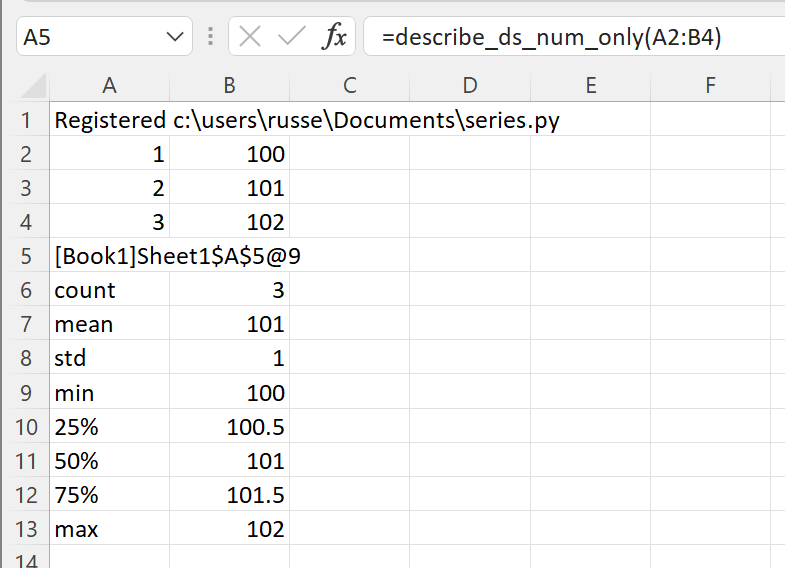The function describe_ds_num_only() behaves exactly the same as describe_ds() however it is far more efficient when passing in a range of numbers.

Note

For optimal performance always use the “_num_only” versions of xlSlim functions when creating numeric pandas Series from Excel Ranges.

## Passing pandas Series to Python functions¶

As shown above, pandas Series can be created from Excel Ranges. xlSlim will create a pandas Series from an Excel Range before passing the pandas Series to the Python function.

Another way to pass pandas Series is to use the cache object handles corresponding to pandas Series in xlSlim’s memory cache.

Let’s create a Series using the create_random_ds() function we defined before.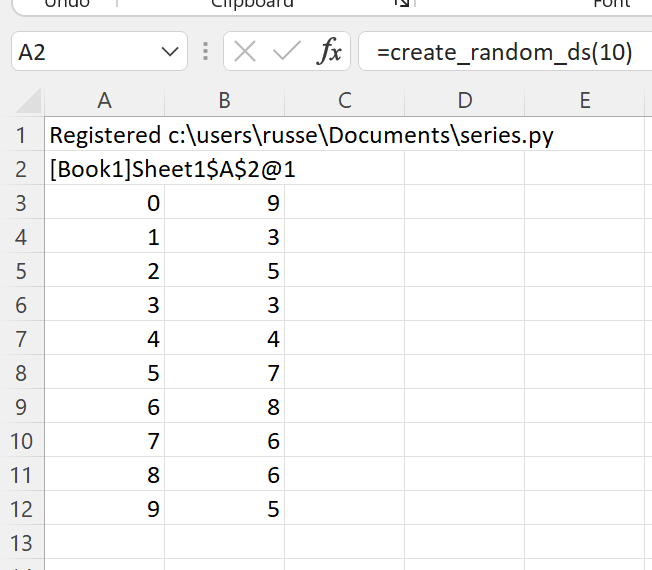The `ViewPyObject()` in A3 shows the Series.

Remove the `ViewPyObject()` function and replace it with the describe_ds_from_handle() function

```=describe_ds_from_handle(A2)
```

This takes in the cache object handle from A2, gets the associated pandas Series from xlSlim’s memory cache, passes the pandas Series to the describe_ds() Python function, and finally stores the result in the memory cache passing a cache object handle back to Excel.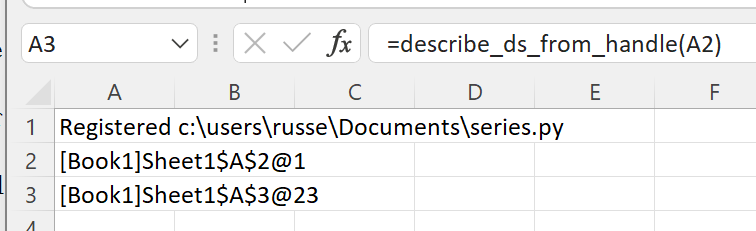The pandas Series describing the object referred to in A2 can be viewed with `ViewPyObject()` as usual.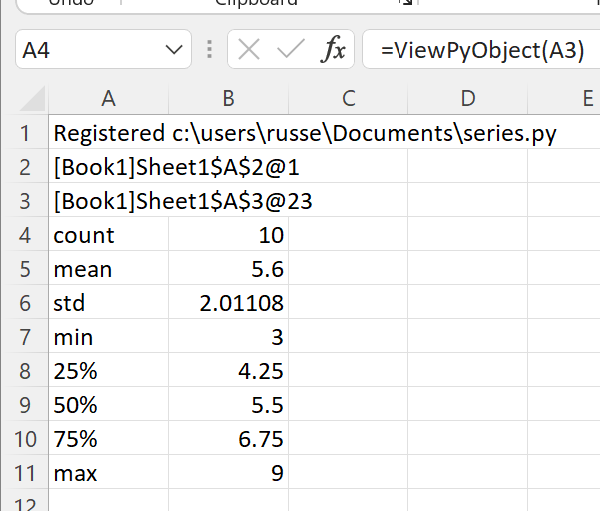Note

Passing pandas Series using cache object handles is much more efficient than creating pandas Series from Excel Ranges.

## Passing multiple pandas Series to Python functions¶

Multiple pandas Series can be passed to Python functions in two ways:
• As separate arguments as shown in the concat_two_ds() function below
• As a list of numpy arrays as shown in the concat_ds() function below
```from typing import List
XLSLIM_INWINDOWFUNC = None

def concat_two_ds(
ds_a: pd.Series,
ds_b: pd.Series,
axis: Optional[int] = 0,
join: Optional[str] = "outer",
ignore_index: Optional[bool] = False,
) -> pd.Series:
"""Concatenate the two supplied Series."""
return concat_df([ds_a, ds_b], axis, join, ignore_index)

def concat_ds(
series: List[pd.Series],
axis: Optional[int] = 0,
join: Optional[str] = "outer",
ignore_index: Optional[bool] = False,
) -> pd.Series:
"""Concatenate the supplied Series."""
# Note how this global function can be used to skip
# running slow code in the Excel function wizard
if XLSLIM_INWINDOWFUNC and XLSLIM_INWINDOWFUNC():
return pd.Series()
return pd.concat(Series, axis=axis, join=join, ignore_index=ignore_index)
```

Given this sheet, where cache object handles in B2 and D2 reference pandas Series created from create_random_ds() and `ViewPyObject()` is used to display the Series at B3 and D3: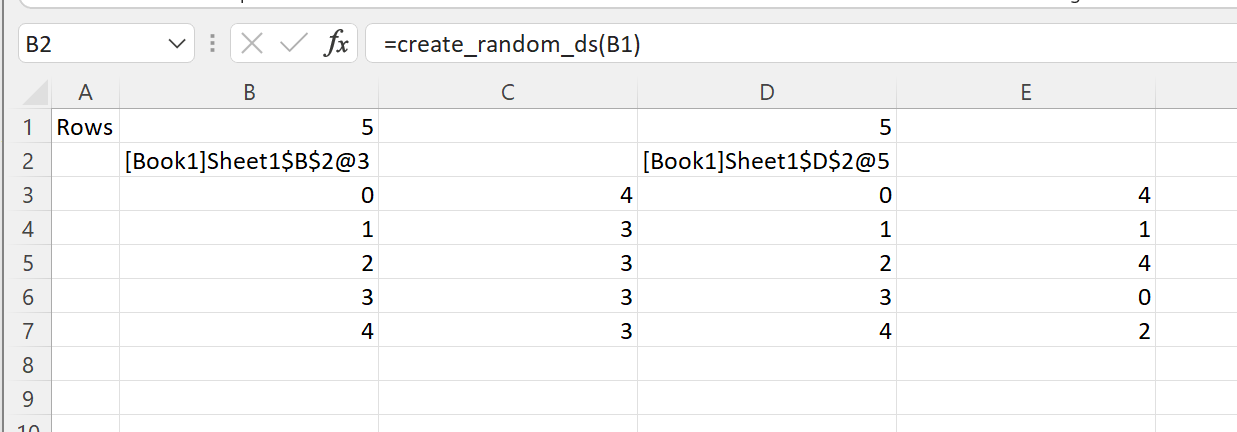For the first case, functions with separate arguments, any of the techniques discussed so far can be use. These are all valid

```=concat_two_ds(B3,D3,,"outer")
=concat_two_ds_num_only(B3,D3,,"outer")
=concat_two_ds_from_handle(B2,D2,,"outer")
```

However for the second case, functions expecting a list, only a list of cache object handles can be supplied.

```=concat_ds(CreateRange(B2,D2),,"outer")
```

We use the `CreateRange()` utility function to create a range as B2 and D2 are not adjacent cells.

Note the use of `XLSLIM_INWINDOWFUNC()` to not concatenate Series if the Excel function wizard is open.